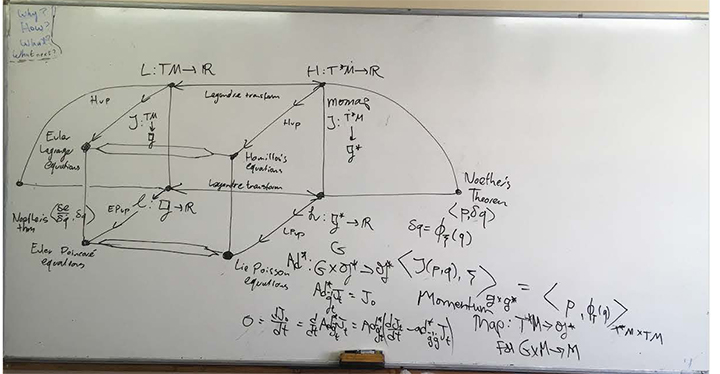Collins Conference Room
Seminar
Speaker:
Darryl D. Holm (Imperial College London)

This event is private.

#### Symmetry and Collective Dynamics

Abstract.  Emmy Noether’s famous theorem (1918) is a cornerstone of theoretical physics. The theorem states that every smooth invertible symmetry of the Lagrangian in Hamil- ton?s variational principle  is associated with a conservation law. For example, momentum and energy are conserved, respectively, for invariance under space and time translations. Another example is the conservation of angular momentum for Lagrangians that are in- variant under rotations. We will explain how the symmetry transformations in Noether?s theorem specify the  optimal variables for expressing  collective  dynamics and  derive  their equations.

This will be a blackboard talk.  I will draw the figure below and explain it, step-by-step.Purpose:
Research Collaboration
SFI Host:
Chris Kempes